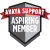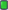Thread: CMS R14 data inconsistency View Single Post
#1
 sciskAspiring MemberJoin Date: May 2016 Posts: 2CMS R14 data inconsistency

Dear all,

I try to design a historical monthly multiagent group report. The problem i have is with below formula which should give 100 (percentage)
column 33
100*((SUM(TOTAL_ACWTIME)+SUM(TOTAL_ACDTIME)+sum(I_ RINGTIME)+sum(TI_AUXTIME)+sum(TI_AVAILTIME)+sum(TI _OTHERTIME))/sum(TI_STAFFTIME))

Above formula was build based on below:
sum(TI_STAFFTIME) = sum(I_ACDTIME + I_ACWTIME + I_DA_ACDTIME + I_DA_ACWTIME + I_RINGTIME + TI_AUXTIME + TI_AVAILTIME + TI_OTHERTIME)

I checked and there is neither I_DA_ACDTIME nor I_DA_ACWTIME reported time.

How it could be that formula from column 33 doesnt give 100 - CMS DB issue?